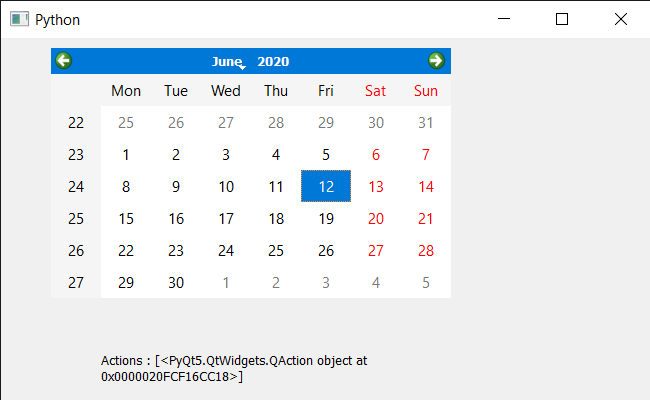Skip to content
Related Articles
PyQt5 QCalendarWidget – Removing QAction
• Last Updated : 15 Jun, 2020

In this article we will see how we can remove the QAction of the QCalendarWidget. In order to do this we use `removeAction` method, this methods emoves the action from this calendar’s list of actions. We can get all the actions with the help of `actions` method.

In order to do this we will use `removeAction` method with the QCalendarWidget object.

Syntax : calendar.removeAction(action)

Argument : It takes QAction object as argument

Return : It return None

Below is the implementation

 `# importing libraries``from` `PyQt5.QtWidgets ``import` `*` `from` `PyQt5 ``import` `QtCore, QtGui``from` `PyQt5.QtGui ``import` `*` `from` `PyQt5.QtCore ``import` `*` `import` `sys`` ` `# QCalendarWidget Class``class` `Calendar(QCalendarWidget):`` ` `    ``# constructor``    ``def` `__init__(``self``, parent ``=` `None``):``        ``super``(Calendar, ``self``).__init__(parent)`` ` ` ` `class` `Window(QMainWindow):`` ` `    ``def` `__init__(``self``):``        ``super``().__init__()`` ` `        ``# setting title``        ``self``.setWindowTitle(``"Python "``)`` ` `        ``# setting geometry``        ``self``.setGeometry(``100``, ``100``, ``650``, ``400``)`` ` `        ``# calling method``        ``self``.UiComponents()`` ` `        ``# showing all the widgets``        ``self``.show()`` ` `    ``# method for components``    ``def` `UiComponents(``self``):`` ` `        ``# creating a QCalendarWidget object``        ``# as Calendar class inherits QCalendarWidget``        ``self``.calendar ``=` `Calendar(``self``)`` ` `        ``# setting geometry to the calender``        ``self``.calendar.setGeometry(``50``, ``10``, ``400``, ``250``)`` ` `        ``# setting cursor``        ``self``.calendar.setCursor(Qt.PointingHandCursor)`` ` `        ``# creating a QAction objects``        ``action1 ``=` `QAction(``"Geek"``, ``self``)``        ``action2 ``=` `QAction(``"Python"``, ``self``)`` ` `        ``# creating action list``        ``action_list ``=` `[action1, action2]`` ` `        ``# adding actions to calendar``        ``self``.calendar.addActions(action_list)`` ` `        ``# removing action``        ``self``.calendar.removeAction(action1)`` ` `        ``# creating label to show the properties``        ``self``.label ``=` `QLabel(``self``)`` ` `        ``# setting geometry to the label``        ``self``.label.setGeometry(``100``, ``280``, ``300``, ``100``)`` ` `        ``# making label multi line``        ``self``.label.setWordWrap(``True``)`` ` `        ``# getting action of the calendar``        ``value ``=` `self``.calendar.actions()`` ` `        ``# setting text to the label``        ``self``.label.setText(``"Actions : "` `+` `str``(value))`` ` ` ` ` ` ` ` `# create pyqt5 app``App ``=` `QApplication(sys.argv)`` ` `# create the instance of our Window``window ``=` `Window()`` ` `# start the app``sys.exit(App.``exec``())`

Output :Attention geek! Strengthen your foundations with the Python Programming Foundation Course and learn the basics.

To begin with, your interview preparations Enhance your Data Structures concepts with the Python DS Course. And to begin with your Machine Learning Journey, join the Machine Learning – Basic Level Course

My Personal Notes arrow_drop_up